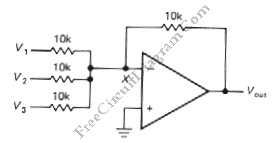# Summing Amplifier with Op-AmpOne of inverting amplifier application is for summing several electric signals. The output of this circuit produce the sums of the input voltages.  We can use both positive or negative voltage for the inputs. The input resistors doesn’t have to be  same values. The input can be expanded to several digits. Since the point X is a virtual ground, the input current is V3/R+V2/R+V1/R. Here is the schematic diagram of the circuit:The Output voltage of this circuit is determined by following equation:
Vout = – (V1 + V2 + V3)
This circuit is usually used as digital-to-analog converter. Using input resistors of   1,25k, 2,5k, 5k, and 10k, the output voltage will represent  binary values 8, 4, 2, and 1.  Sometimes a R-2R ladder circuit is used as input circuit. Another example of this circuit’s application is adding an offset to a signal, or shifting the level for appropriate processing. That’s why this circuit is important in signal conditioning application.